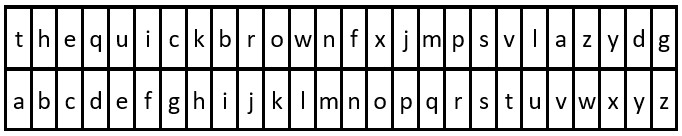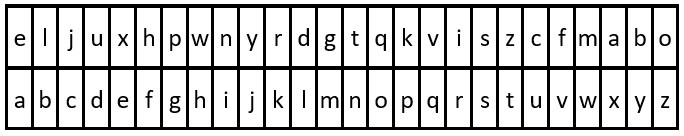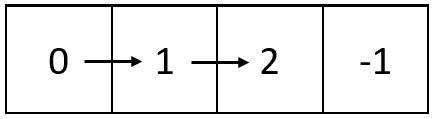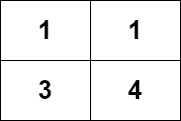# 战局详情

2385 / 6792Juruoer70:29:560:10:570:24:56 1

# 题目及解答

6108. 解密消息

1. 使用 key 中 26 个英文小写字母第一次出现的顺序作为替换表中的字母 顺序

2. 将替换表与普通英文字母表对齐，形成对照表。

3. 按照对照表 替换 message 中的每个字母。

4. 空格 ' ' 保持不变。

• 例如，key = "happy boy"（实际的加密密钥会包含字母表中每个字母 至少一次），据此，可以得到部分对照表（'h' -> 'a''a' -> 'b''p' -> 'c''y' -> 'd''b' -> 'e''o' -> 'f'）。

• 26 <= key.length <= 2000
• key 由小写英文字母及 ' ' 组成
• key 包含英文字母表中每个字符（'a''z'）至少一次
• 1 <= message.length <= 2000
• message 由小写英文字母和 ' ' 组成6111. 螺旋矩阵 IV

• 1 <= m, n <= 1e5
• 1 <= m * n <= 1e5
• 链表中节点数目在范围 [1, m * n]
• 0 <= Node.val <= 10006109. 知道秘密的人数

• 2 <= n <= 1000
• 1 <= delay < forget <= n

• 第 1 天：假设第一个人叫 A 。（一个人知道秘密）
• 第 2 天：A 是唯一一个知道秘密的人。（一个人知道秘密）
• 第 3 天：A 把秘密分享给 B 。（两个人知道秘密）
• 第 4 天：A 把秘密分享给一个新的人 C 。（三个人知道秘密）
• 第 5 天：A 忘记了秘密，B 把秘密分享给一个新的人 D 。（三个人知道秘密）
• 第 6 天：B 把秘密分享给 E，C 把秘密分享给 F 。（五个人知道秘密）

• 第 1 天：第一个知道秘密的人为 A 。（一个人知道秘密）
• 第 2 天：A 把秘密分享给 B 。（两个人知道秘密）
• 第 3 天：A 和 B 把秘密分享给 2 个新的人 C 和 D 。（四个人知道秘密）
• 第 4 天：A 忘记了秘密，B、C、D 分别分享给 3 个新的人。（六个人知道秘密）

• i 天知道秘密的人数 = 第 i - 1 天知道秘密的人数 - 第 i 天新忘记秘密的人数 + 第 i 天新知道秘密的人数。
• i 天新忘记秘密的人数 = 在第 i - forget 天新知道秘密的人数（只有这些人刚好过了 forget 天到达第 i 天）。
• i 天新知道秘密的人数 = 第 i - delay 天所有知道秘密的人数 - 从第 i - delay 天之后所有忘记秘密的人数（因为 forget > delay 所以所有在第 i - delay 天之后忘记秘密的人在第 i - delay 天都记得秘密，而其他没有忘记秘密的人则是第 i 天可以传播秘密的人）。
• i 天为止所有忘记秘密的人数 = 第 i - 1 天所有忘记秘密的人数 + 第 i 天新忘记秘密的人数。

• dp[i] 表示第 i 天知道秘密的人数。
• f[i] 表示到第 i 天为止所有忘记秘密的人数。
• k[i] 表示第 i 天新知道秘密的人数。

• f[i] = f[i - 1] + k[i - forget]
• k[i] = dp[i - delay] - (f[i] - f[i - delay])
• dp[i] = dp[i - 1] - k[i - forget] + k[i]

6110. 网格图中递增路径的数目

• m == grid.length
• n == grid[i].length
• 1 <= m, n <= 1000
• 1 <= m * n <= 1e5
• 1 <= grid[i][j] <= 1e5• 长度为 1 的路径：，，， 。
• 长度为 2 的路径：[1 -> 3]，[1 -> 4]，[3 -> 4] 。
• 长度为 3 的路径：[1 -> 3 -> 4] 。

• 长度为 1 的路径：， 。
• 长度为 2 的路径：[1 -> 2] 。

DFS 记忆化搜索：

dp[i][j] 表示以 grid[i][j] 为终点的路径数，那么可以递归计算 grid[i][j] 四个方向上所有值小于其的格子的路径数。若某个格子的路径数已经计算完毕，则下次递归到它的时候可以直接返回 dp

dp[i][j] 只计算一次，时间复杂度：$O(m \times n)$ ；空间复杂度：$O(m \times n)$